# RD Sharma Solutions For Class 7 Maths Chapter 23 Data Handling - II (Central Values) Exercise 23.3

The PDF of RD Sharma Solutions for Class 7 Exercise 23.3 of Chapter 23 Data Handling – II (Central Values) is given below. Learners can access and download the PDF for free. The solutions to this exercise come with detailed explanations structured by our expert teachers that further makes learning and understanding of concepts an easy task. RD Sharma Solutions for Class 7 textbook includes the concepts in a detailed and step by step way to help the students understand effectively. This exercise includes the median of a group of observations.

## Download the PDF of RD Sharma Solutions For Class 7 Maths Chapter 23 – Data Handling – II (Central Values) Exercise 23.3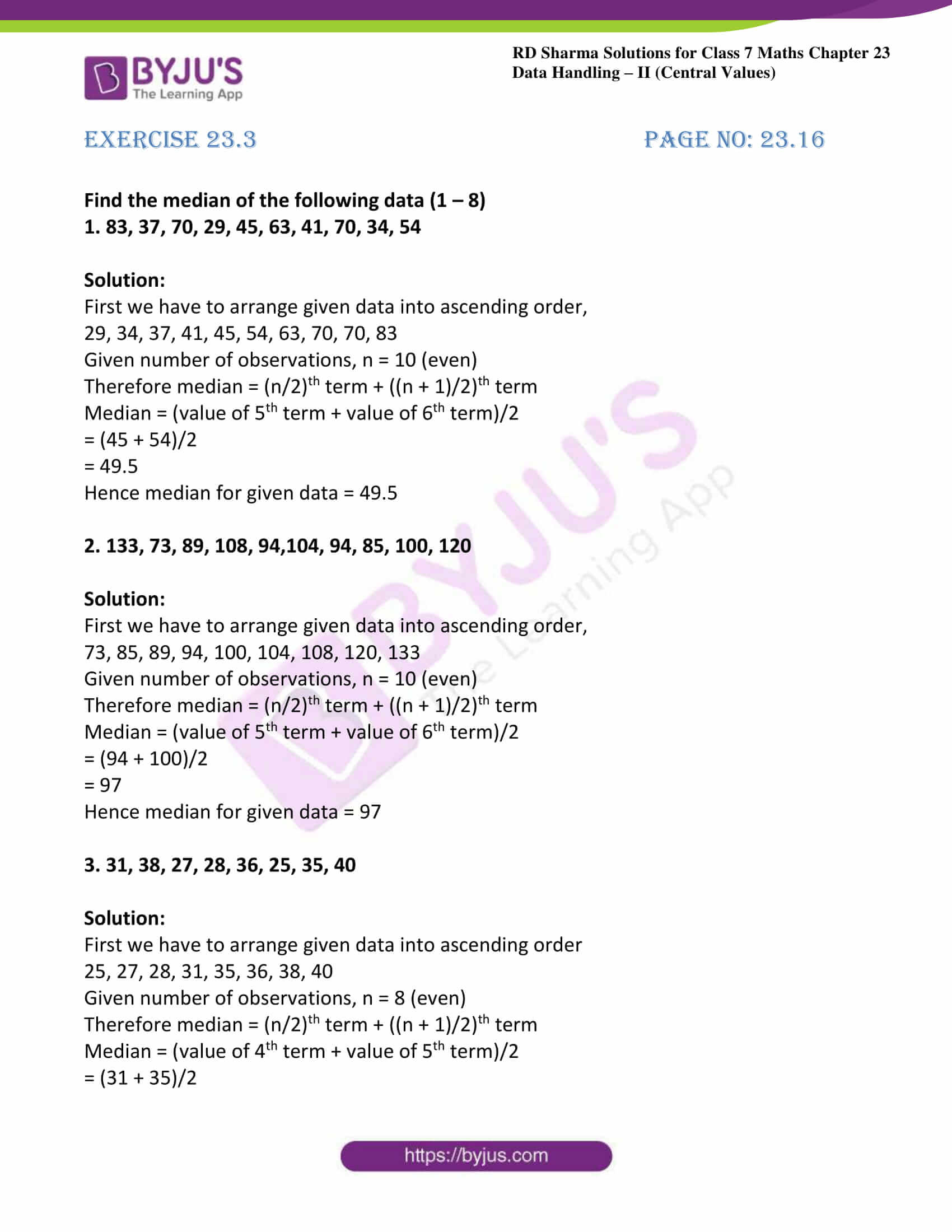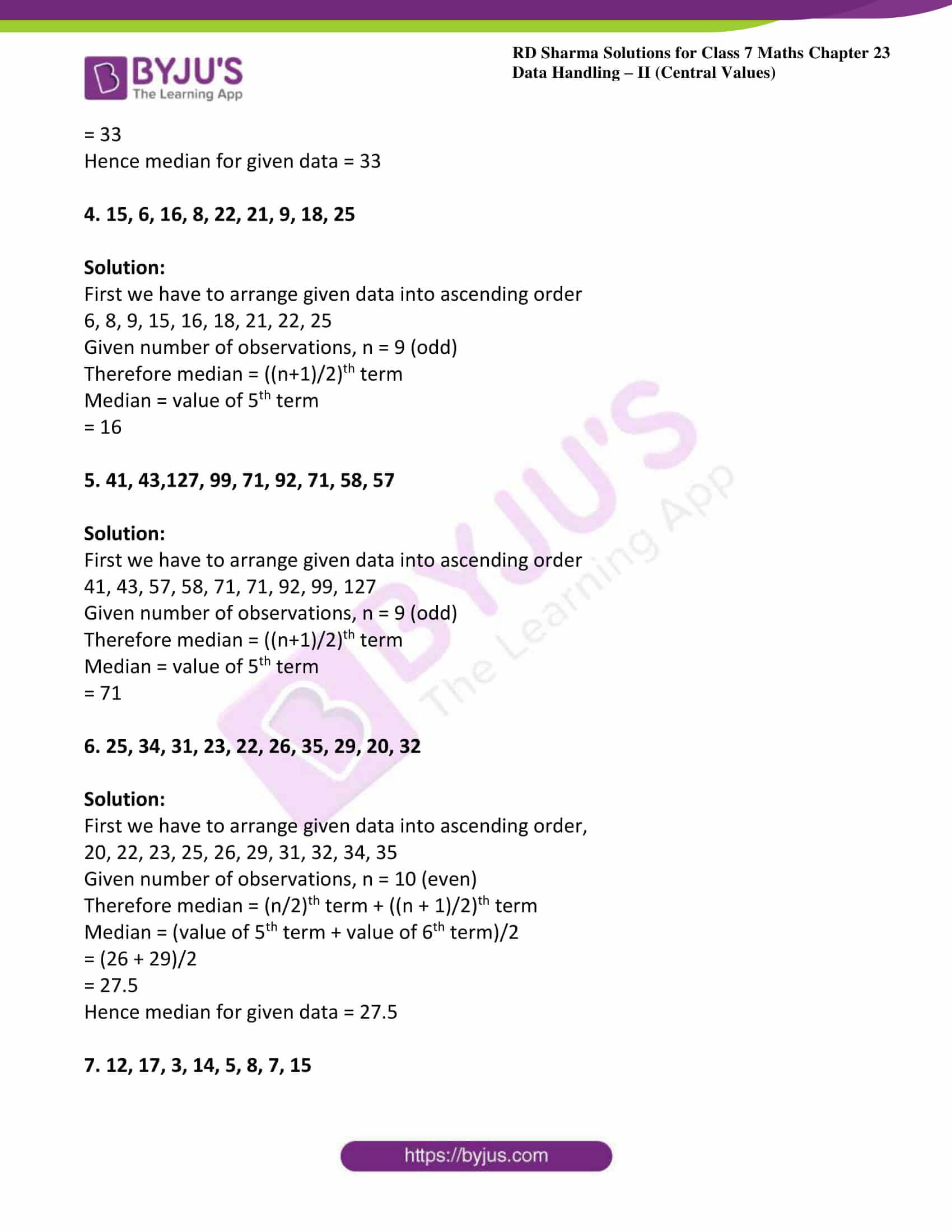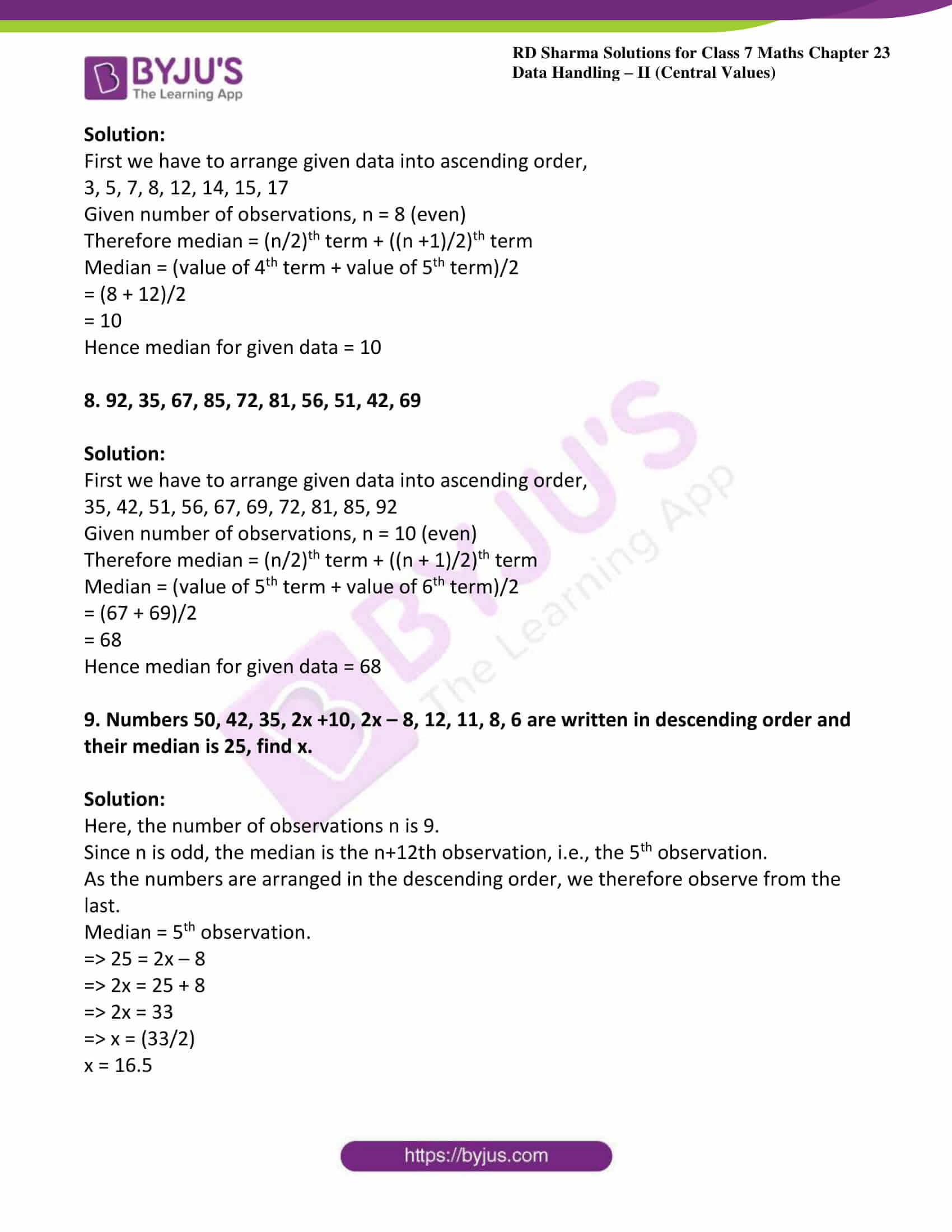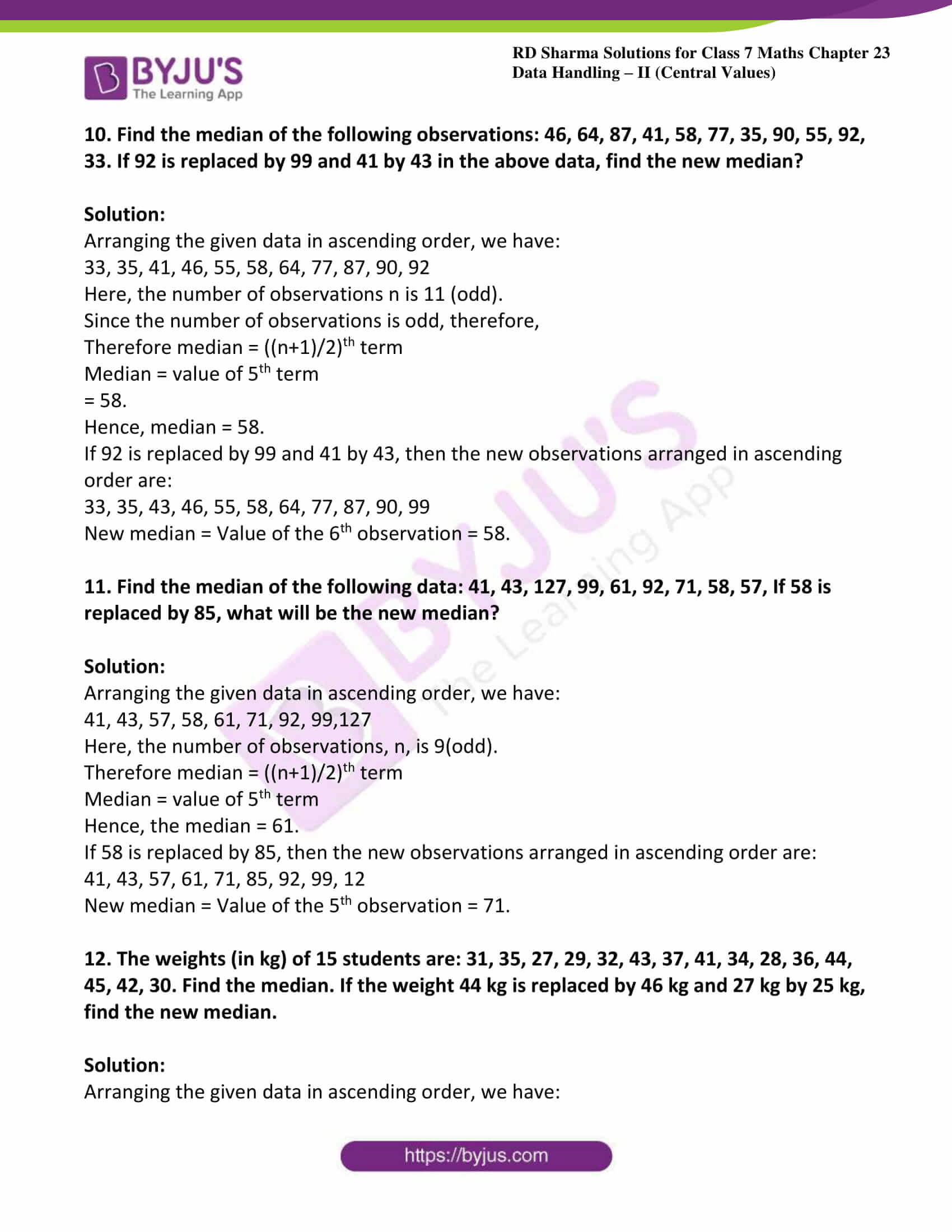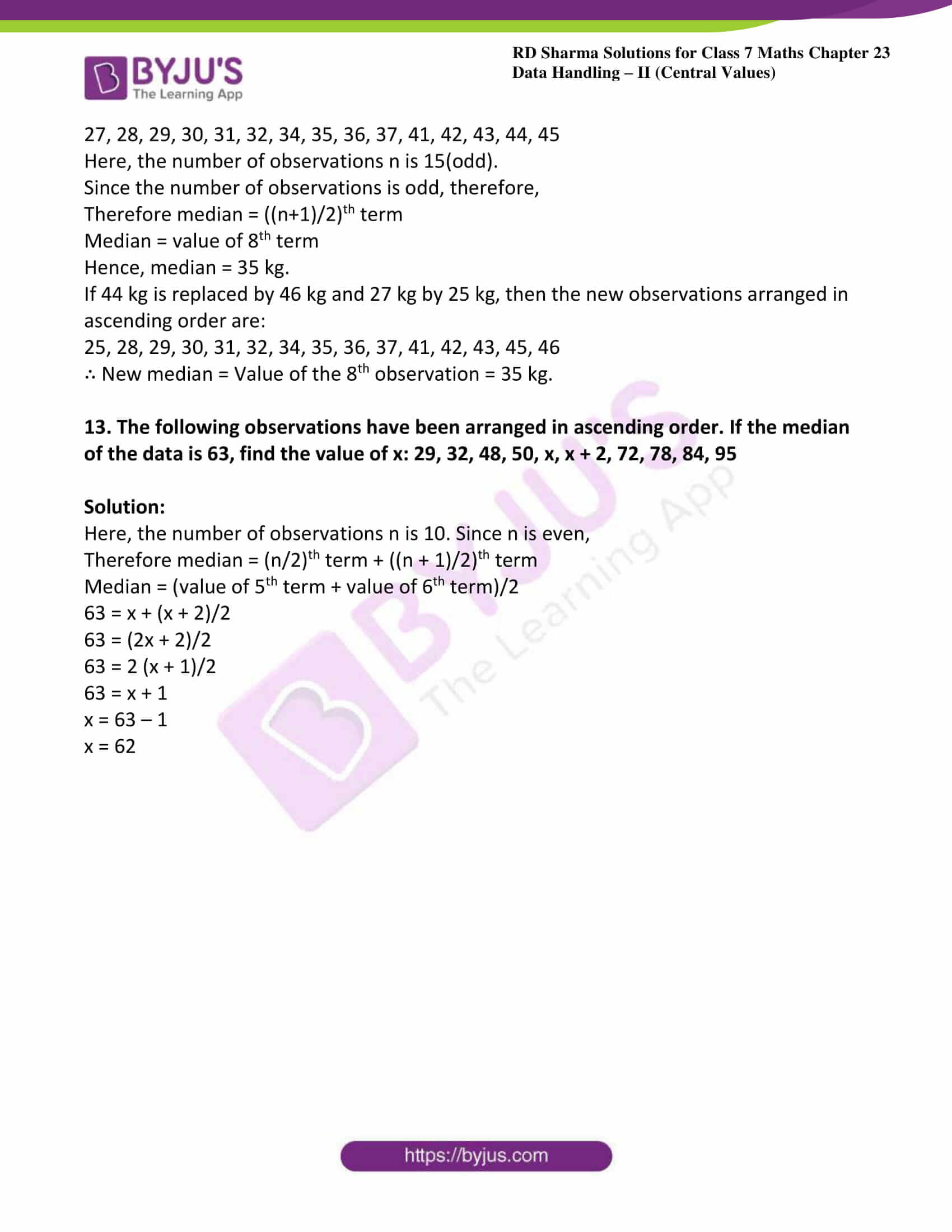### Access answers to Maths RD Sharma Solutions For Class 7 Chapter 23 – Data Handling – II (Central values) Exercise 23.3

Find the median of the following data (1 – 8)

1. 83, 37, 70, 29, 45, 63, 41, 70, 34, 54

Solution:

First we have to arrange given data into ascending order,

29, 34, 37, 41, 45, 54, 63, 70, 70, 83

Given number of observations, n = 10 (even)

Therefore median = (n/2)th term + ((n + 1)/2)th term

Median = (value of 5th term + value of 6th term)/2

= (45 + 54)/2

= 49.5

Hence median for given data = 49.5

2. 133, 73, 89, 108, 94,104, 94, 85, 100, 120

Solution:

First we have to arrange given data into ascending order,

73, 85, 89, 94, 100, 104, 108, 120, 133

Given number of observations, n = 10 (even)

Therefore median = (n/2)th term + ((n + 1)/2)th term

Median = (value of 5th term + value of 6th term)/2

= (94 + 100)/2

= 97

Hence median for given data = 97

3. 31, 38, 27, 28, 36, 25, 35, 40

Solution:

First we have to arrange given data into ascending order

25, 27, 28, 31, 35, 36, 38, 40

Given number of observations, n = 8 (even)

Therefore median = (n/2)th term + ((n + 1)/2)th term

Median = (value of 4th term + value of 5th term)/2

= (31 + 35)/2

= 33

Hence median for given data = 33

4. 15, 6, 16, 8, 22, 21, 9, 18, 25

Solution:

First we have to arrange given data into ascending order

6, 8, 9, 15, 16, 18, 21, 22, 25

Given number of observations, n = 9 (odd)

Therefore median = ((n+1)/2)th term

Median = value of 5th term

= 16

5. 41, 43,127, 99, 71, 92, 71, 58, 57

Solution:

First we have to arrange given data into ascending order

41, 43, 57, 58, 71, 71, 92, 99, 127

Given number of observations, n = 9 (odd)

Therefore median = ((n+1)/2)th term

Median = value of 5th term

= 71

6. 25, 34, 31, 23, 22, 26, 35, 29, 20, 32

Solution:

First we have to arrange given data into ascending order,

20, 22, 23, 25, 26, 29, 31, 32, 34, 35

Given number of observations, n = 10 (even)

Therefore median = (n/2)th term + ((n + 1)/2)th term

Median = (value of 5th term + value of 6th term)/2

= (26 + 29)/2

= 27.5

Hence median for given data = 27.5

7. 12, 17, 3, 14, 5, 8, 7, 15

Solution:

First we have to arrange given data into ascending order,

3, 5, 7, 8, 12, 14, 15, 17

Given number of observations, n = 8 (even)

Therefore median = (n/2)th term + ((n +1)/2)th term

Median = (value of 4th term + value of 5th term)/2

= (8 + 12)/2

= 10

Hence median for given data = 10

8. 92, 35, 67, 85, 72, 81, 56, 51, 42, 69

Solution:

First we have to arrange given data into ascending order,

35, 42, 51, 56, 67, 69, 72, 81, 85, 92

Given number of observations, n = 10 (even)

Therefore median = (n/2)th term + ((n + 1)/2)th term

Median = (value of 5th term + value of 6th term)/2

= (67 + 69)/2

= 68

Hence median for given data = 68

9. Numbers 50, 42, 35, 2x +10, 2x – 8, 12, 11, 8, 6 are written in descending order and their median is 25, find x.

Solution:

Here, the number of observations n is 9.

Since n is odd, the median is the n+12th observation, i.e., the 5th observation.

As the numbers are arranged in the descending order, we therefore observe from the last.

Median = 5th observation.

=> 25 = 2x – 8

=> 2x = 25 + 8

=> 2x = 33

=> x = (33/2)

x = 16.5

10. Find the median of the following observations: 46, 64, 87, 41, 58, 77, 35, 90, 55, 92, 33. If 92 is replaced by 99 and 41 by 43 in the above data, find the new median?

Solution:

Arranging the given data in ascending order, we have:

33, 35, 41, 46, 55, 58, 64, 77, 87, 90, 92

Here, the number of observations n is 11 (odd).

Since the number of observations is odd, therefore,

Therefore median = ((n+1)/2)th term

Median = value of 5th term

= 58.

Hence, median = 58.

If 92 is replaced by 99 and 41 by 43, then the new observations arranged in ascending order are:

33, 35, 43, 46, 55, 58, 64, 77, 87, 90, 99

New median = Value of the 6th observation = 58.

11. Find the median of the following data: 41, 43, 127, 99, 61, 92, 71, 58, 57, If 58 is replaced by 85, what will be the new median?

Solution:

Arranging the given data in ascending order, we have:

41, 43, 57, 58, 61, 71, 92, 99,127

Here, the number of observations, n, is 9(odd).

Therefore median = ((n+1)/2)th term

Median = value of 5th term

Hence, the median = 61.

If 58 is replaced by 85, then the new observations arranged in ascending order are:

41, 43, 57, 61, 71, 85, 92, 99, 12

New median = Value of the 5th observation = 71.

12. The weights (in kg) of 15 students are: 31, 35, 27, 29, 32, 43, 37, 41, 34, 28, 36, 44, 45, 42, 30. Find the median. If the weight 44 kg is replaced by 46 kg and 27 kg by 25 kg, find the new median.

Solution:

Arranging the given data in ascending order, we have:

27, 28, 29, 30, 31, 32, 34, 35, 36, 37, 41, 42, 43, 44, 45

Here, the number of observations n is 15(odd).

Since the number of observations is odd, therefore,

Therefore median = ((n+1)/2)th term

Median = value of 8th term

Hence, median = 35 kg.

If 44 kg is replaced by 46 kg and 27 kg by 25 kg, then the new observations arranged in ascending order are:

25, 28, 29, 30, 31, 32, 34, 35, 36, 37, 41, 42, 43, 45, 46

∴ New median = Value of the 8th observation = 35 kg.

13. The following observations have been arranged in ascending order. If the median of the data is 63, find the value of x: 29, 32, 48, 50, x, x + 2, 72, 78, 84, 95

Solution:

Here, the number of observations n is 10. Since n is even,

Therefore median = (n/2)th term + ((n + 1)/2)th term

Median = (value of 5th term + value of 6th term)/2

63 = x + (x + 2)/2

63 = (2x + 2)/2

63 = 2 (x + 1)/2

63 = x + 1

x = 63 – 1

x = 62